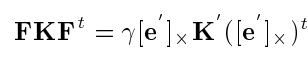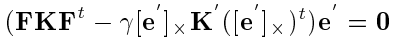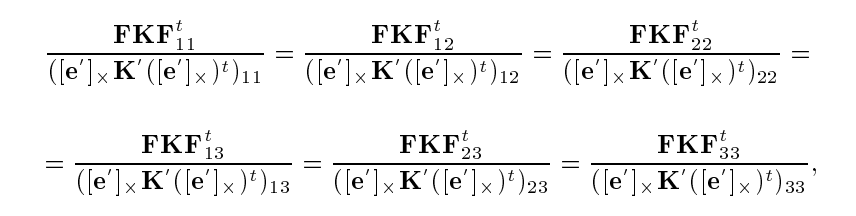# Methods

Our methodology involves the use of the Structure-from-Motion (SfM) and other external optimization strategies from Colmap to estimate the camera intrinsics.

We input camera extrinsic and images captured from various viewpoints and obtain improved camera intrinsics parameters as output. Merely relying on the Structure-from-Motion approach does not yield sufficiently accurate camera intrinsic values. Hence, we incorporate Kruppa equations for self-calibration to acquire an initial camera intrinsics estimate, which we then utilize as an input to our pipeline.

## Structure-from-Motion Pipeline:

The SfM pipeline proceeds as follows:

• Image features are extracted from every image and matched to identify the overlapping part of the scene between every image pair
• 3D world coordinates and camera poses are obtained through the triangulation of matched points in each image pair. New points and matches are incrementally added with each additional image.
• Bundle adjustment refines 3D point coordinates and camera poses by minimizing the reprojection error. The accuracy of the 3D model is progressively improved as more images are added and the bundle adjustment is iteratively applied.

## Kruppa equations

Kruppa’s equations, which are widely known as the first camera self-calibration method were first developed and used in the early 2000s. However, these equations are extremely sensitive to noise.

Given,

• F – Fundamental Matrix between any camera pair
• A, A’ – Unknown intrinsics of the camera pair
• K = AA^T

We use the relationship between the essential matrix and fundamental matrix to eliminate all the unknowns and establish a relationship between the Fs and Ks of the camera pairs. This gives us 6 equations per camera pair out of which only 2 of them are linearly independent. Hence, for m cameras, we will have m*(m-1) equations to solve for 4*m unknowns (camera intrinsics – fx, fy, cx, cy).Classical Kruppa Equations –